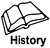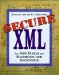# 13.1 The KeyValue Element

The KeyValue element contains the actual value of a single public key that may be useful in validating a signature, decrypting data, or agreeing on a key. See Table 13-1 for its identifier. The following sections specify structures for DSA, RSA, and DH keys. The KeyValue element can in clude application-defined keys as element content qualified by another namespace or as character content. Element content is the preferred method because namespaces provide a mechanism to avoid conflicts.

XMLDSIG defines the KeyValue element syntax as follows:

` <!-- XMLDSIG KeyValue DTD --> <!ELEMENT KeyValue (#PCDATA|DSAKeyValue|RSAKeyValue                     %KeyValue.ANY;)* > `

As expanded by XML Encryption, it would be something like this:

` <!-- Extended KeyValue DTD --> <!ELEMENT KeyValue (#PCDATA|DSAKeyValue|RSAKeyValue|DHKeyValue                     %KeyValue.ANY;)* > `

In schema notation, the extended syntax has the following form:

` <!-- Extended KeyValue schema --> <element name="KeyValue" type="ds:KeyValueType"/> <complexType name="KeyValueType" mixed="true">     <choice>         <element ref="ds:DSAKeyValue"/>         <element ref="ds:RSAKeyValue"/>         <element ref="enc:DHKeyValue"/>         <any namespace="##other" processContents="lax"/>     </choice> </complexType> `

Table 13-2 lists the child elements of KeyValue (grandchild elements of KeyInfo) specified by XML Security. You use the listed URI in the Type attribute of RetrievalMethod or Reference elements.When XMLDSIG standardization was initiated, the RSA patent had not yet expired. IETF policy stated that DSA signatures were the preferred, mandatory-to-implement algorithm. (Having one or more mandatory-to-implement algorithms is necessary to ensure that different implementations can interoperate.) RSA, as encumbered technology, could only be recommended. Since then, the RSA patent has expired. Newer IETF standards frequently mandate RSA and make DSA only recommended or optional, although sometimes both are mandatory; however, particularly with XMLDSIG going jointly through both the IETF and W3C standards processes, it is bureaucratically difficult to go back and change such things. All known XMLDSIG implementations include RSA signature support, so you will be reasonably safe if you think of both as required.

##### Table 13-2. KeyValue Child Elements
Implementation Element Name Type URI

XMLDSIG: Required XMLENC: N/A

DSAKeyValue http://www.w3.org/2000/09/xmldsig#DSAKeyValue

XMLDSIG: Recommended

XMLENC: Required

RSAKeyValue http://www.w3.org/2000/09/xmldsig#

XMLDSIG: Optional

XMLENC: Optional

DHKeyValue http://www.w3.org/2001/04/xmlenc#DHKeyValue

#### 13.1.1 The DSAKeyValue Element

[FIPS 186-2] specifies Digital Signature Algorithm (DSA) keys and the DSA. DSA public key values can have the following fields:

• P = a prime modulus meeting the [FIPS 186-2] requirements

• Q = an integer in the range 2159 < Q < 2160, which is a prime divisor of P-1

• G = an integer with certain properties with respect to P and Q

• J = (P - 1) / Q

• Y = GX mod P (where X is part of the private key and not made public)

• seed = a DSA prime generation seed

• pgenCounter = a DSA prime generation counter

Parameter J is available for inclusion solely for efficiency, as it is calculable from P and Q. Parameters seed and pgenCounter are used in the DSA prime number generation algorithm specified in [FIPS 186-2]. As such, they are optional; note, however, that either both must be present or both must be absent. This prime generation algorithm, which provides assurance that a weak prime is not being used, yields values for P and Q.

Parameters P, Q, and G can be public and common to a group of users. They might be known from the application context. As such, they are optional; note, however, that P and Q must either both appear or both be absent. If P, Q, seed, and pgenCounter are all present, implementations are not required to check whether they are consistent and are free to use either P and Q or seed and pgenCounter. All parameters are encoded as base-64 [RFC 2045] values.

Arbitrary-length integers (e.g., "bignums" such as RSA moduli) are represented in XML octet strings as defined by the XML digital signature CryptoBinary type (see Chapter 10).

The U.S. Digital Signature Algorithm [FIPS 186-2] uses DSA keys. The DTD for a DSAKeyValue is as follows:

` <!-- DSAKeyValue DTD --> <!ELEMENT DSAKeyValue (P, Q)?, J?, G?, Y,                       (Seed, PgenCounter)?) > <!ELEMENT P (#PCDATA) > <!ELEMENT Q (#PCDATA) > <!ELEMENT J (#PCDATA) > <!ELEMENT G (#PCDATA) > <!ELEMENT Y (#PCDATA) > <!ELEMENT Seed (#PCDATA) > <!ELEMENT PgenCounter (#PCDATA) > `

In schema notation, it has the following form:

` <!-- DSAKeyValue Schema --> <element name="DSAKeyValue" type="ds:DSAKeyValueType"/> <complexType name="DSAKeyValueType">     <sequence>         <sequence minOccurs="0">             <element name="P" type="ds:CryptoBinary"/>             <element name="Q" type="ds:CryptoBinary"/>         </sequence>         <element name="J" type="ds:CryptoBinary"                  minOccurs="0"/>         <element name="G" type="ds:CryptoBinary"                  minOccurs="0"/>         <element name="Y" type="ds:CryptoBinary"/>         <sequence minOccurs="0">             <element name="Seed" type="ds:CryptoBinary"/>             <element name="PgenCounter"                      type="ds:CryptoBinary"/>         </sequence>     </sequence> </complexType> `

#### 13.1.2 The RSAKeyValue Element

RSA key values have two fields Modulus and Exponent. Here is an example:

` <RSAKeyValue> <Modulus> xA7SEU+e0yQH5rm9kbCDN9o3aPIo7HbP7tX6WoocLZ     AtNfyxSZDU16ksL6WjubafOqNEpcwR3RdFsT7bCqnXPBe5ELh5u4     VEy19MzxkXRgrMvavzyBpVRgBUwUlV5foK5hhmbktQhyNdy/6LpQ     RhDUDsTvK+g9Ucj47es9AQJ3U= </Modulus> <Exponent>AQAB</Exponent> </RSAKeyValue> `

XML Security arbitrary-length integers (e.g., "bignums" such as RSA moduli) are represented as octet strings as defined by the digital signature CryptoBinary type (see Chapter 10).

The Rivest-Shamir-Adelman algorithm [RSA] uses RSA keys. You can use this algorithm for both signing and encryption. The DTD for an RSAKeyValue element follows:

` <!-- RSAKeyValue DTD --> <!ELEMENT RSAKeyValue (Modulus, Exponent) > <!ELEMENT Modulus (#PCDATA) > <!ELEMENT Exponent (#PCDATA) > `

In schema notation, it has the following form:

` <!-- RSAKeyValue Schema --> <element name="RSAKeyValue"          type="ds:RSAKeyValueType"/> <complexType name="RSAKeyValueType">     <sequence>         <element name="Modulus"                  type="ds:CryptoBinary"/>         <element name="Exponent"                  type="ds:CryptoBinary"/>     </sequence> </complexType> `

#### 13.1.3 The DHKeyValue Element

A Diffie-Hellman (DH) public key, as specified in [RFC 2631], consists of a maximum of six quantities: two large primes ("P" and "Q"), a "Generator" (g), the public key ("Public"), and two validation parameters ("seed" and "pgenCounter"). These quantities are related as follows:

• Public = (gx mod p), where x is the corresponding private key

• P = (j * q + 1) where j >= 2

The "seed" and "pgenCounter" values are optional and can be used to determine whether the DH key has been generated in conformance with the algorithm specified in [RFC 2631]. Because the primes and Generator can be safely shared over many DH keys, they may be known from the application environment and are optional.

The DTD for a DHKeyValue follows:

` <!-- DHKeyValue DTD --> <!ELEMENT DHKeyValue (( P, Q, Generator)?, Public,                       ( seed, pgenCounter)?) > <!ELEMENT P (#PCDATA) > <!ELEMENT Q (#PCDATA) > <!ELEMENT Generator (#PCDATA) > <!ELEMENT Public (#PCDATA) > <!ELEMENT seed (#PCDATA) > <!ELEMENT pgenCounter (#PCDATA) > `

In schema notation, it has the following form:

` <!-- DHKeyValue Schema --> <element name="DHKeyValue"          type="enc:DHKeyValueType"/>    <complexType name="DHKeyValueType">       <sequence>          <sequence minOccurs="0">             <element name="P"                      type="ds:CryptoBinary"/>             <element name="Q"                      type="ds:CryptoBinary"/>             <element name="Generator"                      type="ds:CryptoBinary"/>          </sequence>          <element name="Public"                   type="ds:CryptoBinary"/>          <sequence minOccurs="0">             <element name="seed"                      type="ds:CryptoBinary"/>             <element name="pgenCounter"                      type="ds:CryptoBinary"/>          </sequence>       </sequence>    </complexType> </element> `Secure XML: The New Syntax for Signatures and Encryption
ISBN: 0201756056
EAN: 2147483647
Year: 2005
Pages: 186